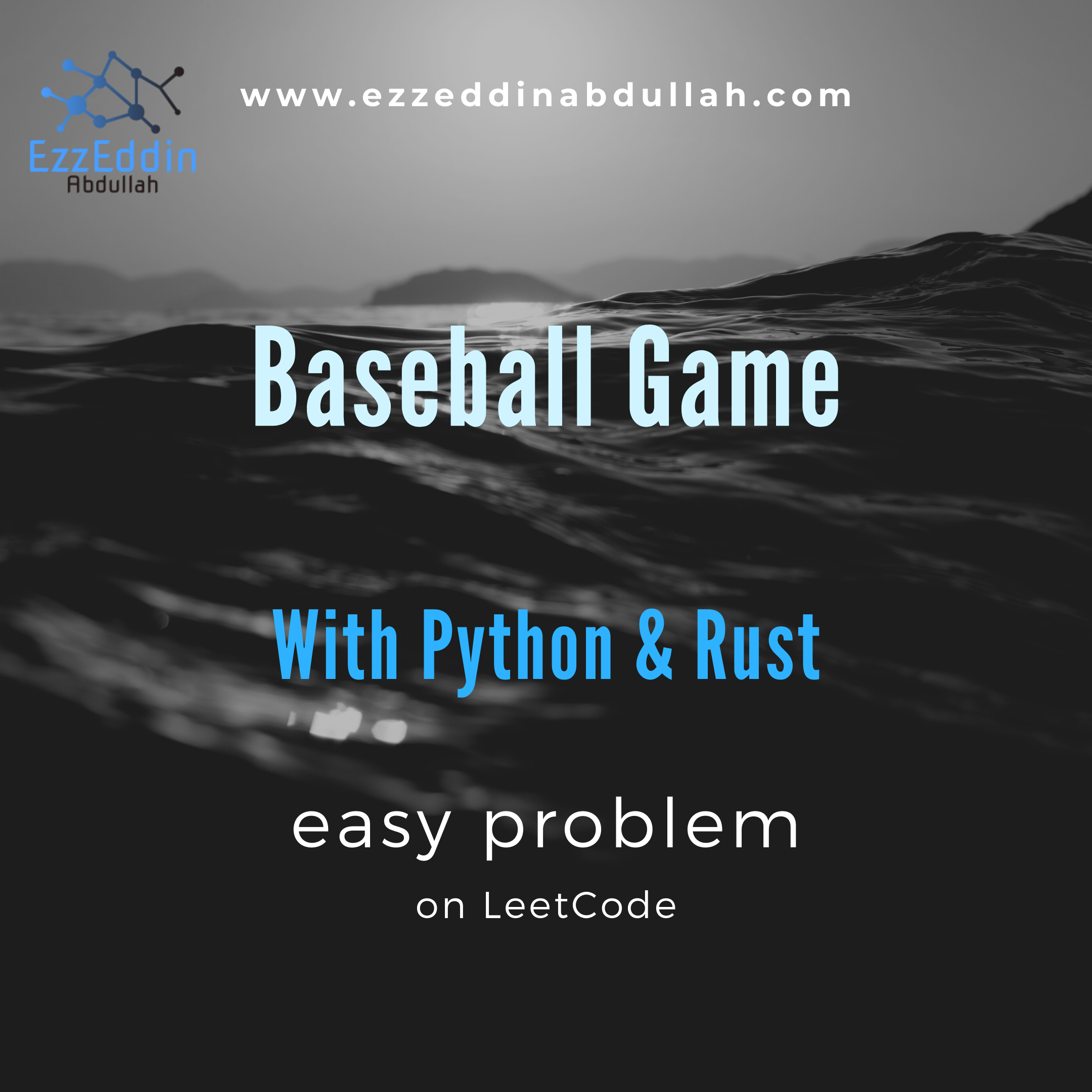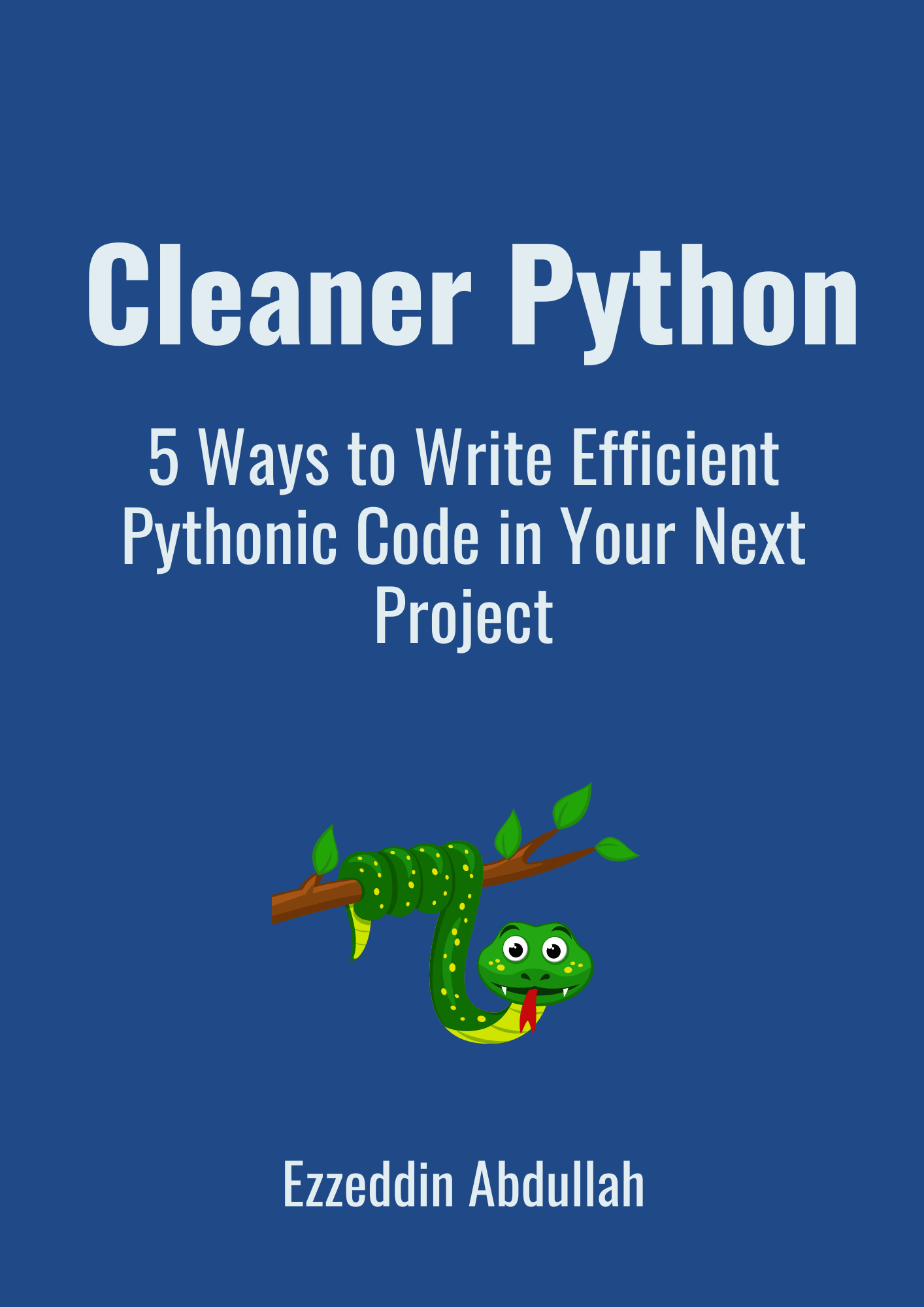# Baseball Game With Python and Rust

Learn the fundamentals of programming by asking questionsWhat programmers do when they solve a problem is that they convert the logic to questions and then try to find answers to those questions.

We always do these steps in any programming problem:

1. Convert the business logic to questions
2. Be able to find answers to these questions in code

And will go through each line of each code written in Python and Rust to be able to answer the questions raised.

Let’s do it

## The game

Baseball Game is an easy problem at leetcode, I hope you have read the problem and tried to solve it. In this game, you’re given an array of strings of operations and it’s required to calculate the sum of the elements of the final array. But what are these operations?

These operations are one of the following:

• A number: which should be stored in an array
• D: to double the last element in the array
• C: to remove the last element from the array
• +: to add the last two elements of the array

## Manipulating an array

So we’re given an array of strings and we’d like to manipulate a different array of integers in such a way it gets updated with the integer elements ending up with their sum as the output

We need to ask ourselves these questions to solve this game:

1. How to store the input array of strings?
2. How to create an array to store integers?
3. How to loop over an array?
4. How to check if an element in the array equals “D” or “C” etc?
5. How to convert a string into an integer?
6. How to get the last two elements in the array?
7. How to add an element at the end of the array?
8. How to remove the last element from the array?
9. How to sum the elements in the array?

Let’s answer these questions by going through the codes below, line by line for each language: Python and Rust

Hint: skip the part of Python if you’re comfortable with it, Rust is in the next section

## Python

1. How to store the input array of strings?

### ops = ["5","2","C","D","+"]

An array in Python is called a list so here we can store a list of strings as if we store numeric elements. The only difference is that each element is enclosed by double-quotes.

2. How to create an array to store integers?

### record = []

In Python initializing a list is as simple as assigning the empty list represented by square brackets to a new variable record here.

3. How to loop over an array?

### for op in ops:

Now, this is one type of loop in Python which is a for loop which iterates over the elements of ops array so each element in the array is represented by op.

4. How to check if an element in the array equals “D” or “C” etc?

### if op == "D":

Creating a condition if D character is in the array but we’d like to also check if there is a C character existing, so we make an else if statement which is elif in Python:

### elif op == "C":

What if there is a plus sign:

### elif op == "+":

What if there is a condition nothing like the others listed above?

### else:

which should be integers in our case and we’re sure about that from the problem at leetcode. Other than that, we should make another condition with elif to make sure about the possibilities of the user input.

5. How to convert a string into an integer?

### int(<string>)

In Python, casting a string into an integer is as easy as putting the string into the int() function.

6. How to get the last two elements in the array?

An easy way to get the elements from the end is to put a negative index in the array, so this:

### record[-1]

will get you the last element

and this:

### record[-2]

will output the element before the last

7. How to add an element to the end of the array?

using the append() method in Python like these statements:

### record.append(2 * int(record[-1]))

to append double the last element to the array

and

### record.append(int(record[-1]) + int(record[-2]))

to append the sum of the last two elements

and

### record.append(int(op))

to append the integer element

8. How to remove the last element from the array?

Removing the element from the end of the list in Python is done by popping it from the list using pop() method like this:

### record.pop()

to remove the last element when we see the character C

9. How to sum the elements in the array?

as simple as using the function sum() to the array

## Rust

1. How to store the input array of strings?

### let ops: Vec<String> = vec!["5".to_string(), "2".to_string(), "C".to_string(), "D".to_string(), "+".to_string()];

In Rust, storing a vector of strings is not as straightforward as Python’s so here we need to convert each element to a string first using the to_string() method and apply it to every element separated by commas and put them into a vector with a type of String.

Assigning a variable in Rust starts with the let keyword and by default, this variable is immutable meaning it can’t be modified which is obvious in this example because we want to keep the input unchanged and pass it to cal_points() the function we made.

The data type is specified when calling assigning a variable in Rust with Vec<String> to be a vector of strings and this vector is initiated by vec! before the square brackets.

2. How to create an array to store integers?

### let mut record: Vec<i32> = Vec::new();

As Rust is a statically typed language meaning it must know all types of variables at compile time, initializing a vector is like the above with specifying the size of the vector and its type. The size here is a signed number of 32bit which means you can put values in that vector with a minimum of -2³¹ until a max value of 2³¹ — 1

3. How to loop over an array?

### for op in ops {<statement(s)>}

Now, this is one type of loop in Rust which is a for loop which iterates over the elements of ops array so each element in the array is represented by op, and the statements are enclosed between the curly braces.

4. How to check if an element in the array equals “D” or “C” etc?

### if op == "D" {<statement(s)}

Creating a condition if D character is in the array but we’d like to also check if there is a C character existing, so we make an else if statement which is the same else if in Rust:

### else if op == "C" {<statement(s)>}

What if there is a plus sign:

### else if op == "+" {<statement(s)>}

What if there is a condition nothing like the others listed above?

### else {<statement(s)>}

which should be integers in our case and we’re sure about that from the problem at leetcode. Other than that, we should make another condition with another else if to make sure about the possibilities of the user input.

5. How to convert a string into an integer?

### op.parse::<i32>().unwrap()

In Rust, casting a string into an integer is specified by the type of integer you’re converting to which can be done by parsing the signed integer  enclosed by the turbofish operator ::<> and using unwrap() method which passes this converted integer as is except if this value is None.

6. How to get the last two elements in the array?

To get the elements from the end of a vector, put the index of the same size of the vector minus 1 and then subtract it by 1 in order to get the element before it (or the element after the other starting from the right of the vector) so:

### record[record.len()-1]

will get you the last element

and this:

### record[record.len()-2]

will output the element before the last

7. How to add an element to the end of the array?

using the push() method in Rust like these statements:

### record.push(2 * record[record.len()-1]);

to append double the last element to the array

and

### record.push(record[record.len()-1] + record[record.len()-2])

to append the sum of the last two elements

and

### record.push(op.parse::<i32>().unwrap());

to append the integer element

8. How to remove the last element from the array?

Removing the element from the end of the vector in Rust is done as in Python using pop() method like this:

### record.pop()

to remove the last element when we see the character C

9. How to sum the elements in the array?

In Rust, you have to convert the vector to an iterator in which you can apply the sum method to it with a turbofish operator of the signed 32bit number

## Final thoughts

We’ve seen how to store an array of strings, how to initialize and loop over it, how to create a condition, how to convert a string into an integer, how to get and remove the last elements and add elements in an array, and how to sum the elements in an array.

We’ve done this line by line for a specific case study for two programming languages: Python and Rust

Published on my new medium publication, make sure to follow it: Brainwave